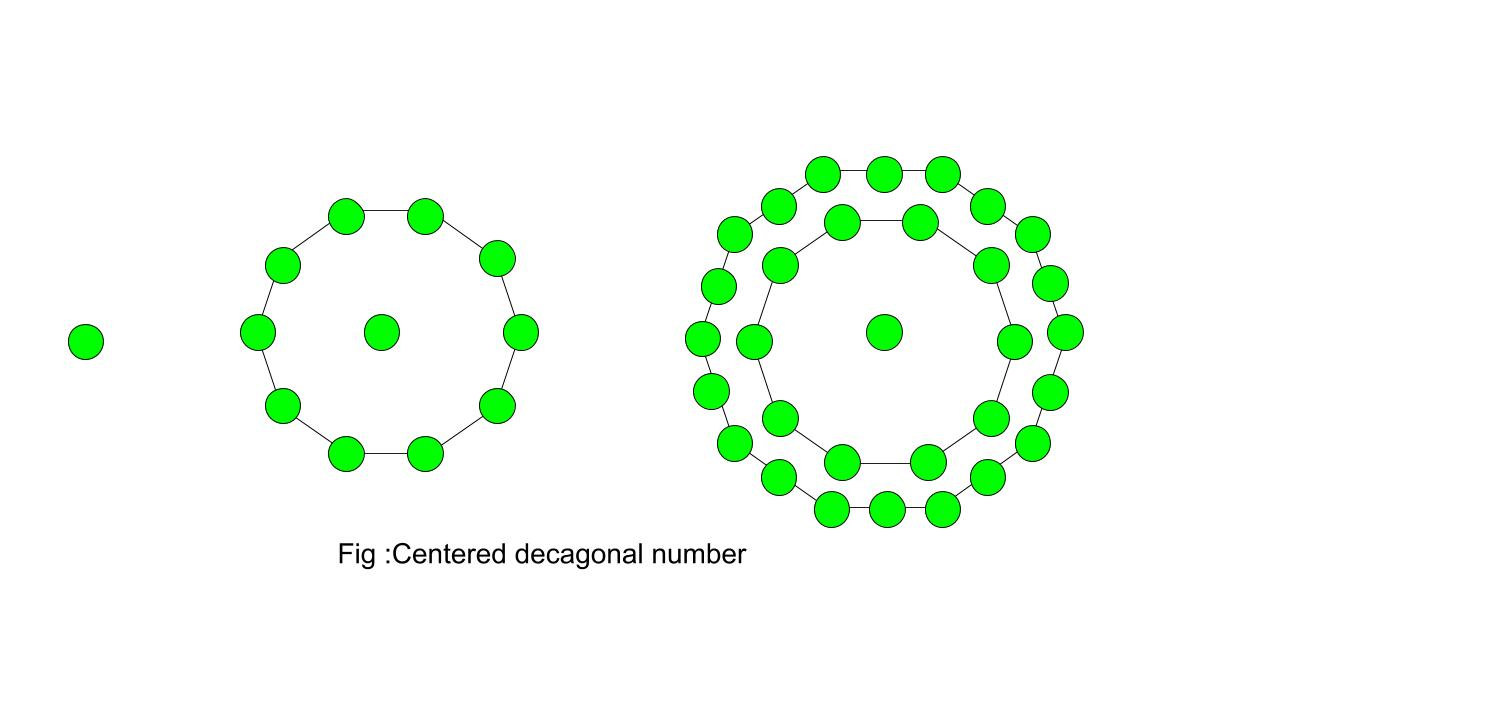# Centered decagonal number

Given a number n, find the nth Centered decagonal number .

A Centered Decagonal Number is centered figurative number that represents a decagon with dot in center and all other dot surrounding it in successive decagonal form. Source[Wiki].The first few Centered Decagonal Numbers are :
1, 11, 31, 61, 101, 151, 211, 281, 361, 451, 551, 661…………

Examples :

```Input :  3
Output : 31

Input : 6
Output : 151
```

## Recommended: Please try your approach on {IDE} first, before moving on to the solution.

In mathematics centered decagonal number for n-th term is given by :

``````

Below is the basic implementation of the above idea.

## C++

 `// Program to find nth ` `// centered decagonal  ` `// number ` `#include ` `using` `namespace` `std; ` ` `  `// Centered decagonal ` `// number function ` ` `  `int` `centereddecagonalnum(``int` `n) ` `{ ` `    ``// Formula to calculate nth ` `    ``// centered decagonal number & ` `    ``// return it into main function. ` `    ``return` `(5 * n * n + 5 * n + 1); ` `} ` ` `  `// Driver Code ` `int` `main() ` `{ ` `    ``int` `n = 5; ` `    ``cout << n << ``"th centered decagonal"` `                          ``<< ``"number: "``; ` `    ``cout << centereddecagonalnum(n); ` `    ``cout << endl; ` `    ``n = 9; ` `    ``cout << n << ``"th centered decagonal"` `                          ``<< ``"number: "``; ` `    ``cout << centereddecagonalnum(n); ` ` `  `    ``return` `0; ` `} `

## Java

 `// Java Program to find nth ` `// centered decagonal number ` `import` `java.io.*; ` ` `  `class` `GFG  ` `{ ` `     `  `    ``// Centered decagonal ` `    ``// number function ` `    ``static` `int` `centereddecagonalnum(``int` `n) ` `    ``{ ` `         `  `    ``// Formula to calculate nth ` `    ``// centered decagonal number & ` `    ``// return it into main function. ` `    ``return` `(``5` `* n * n + ``5` `* n + ``1``); ` `    ``} ` ` `  `    ``// Driver Code ` `    ``public` `static` `void` `main (String[] args)  ` `    ``{ ` `        ``int` `n = ``5``; ` `        ``System.out.print(n + ``"th centered "` `+  ` `                       ``"decagonal number: "``); ` `        ``System.out.println(centereddecagonalnum(n)); ` ` `  `        ``n = ``9``; ` `        ``System.out.print(n + ``"th centered "` `+  ` `                       ``"decagonal number: "``); ` `        ``System.out.println(centereddecagonalnum(n)); ` `    ``} ` `} ` ` `  `// This code is contributed by m_kit `

## Python 3

 `# Program to find nth ` `# centered decagonal number ` ` `  `# Centered decagonal ` `# number function ` `def` `centereddecagonalnum(n) : ` `     `  `    ``# Formula to calculate  ` `    ``# nth centered decagonal  ` `    ``# number & return it ` `    ``# into main function. ` `    ``return` `(``5` `*` `n ``*` `n ``+`  `            ``5` `*` `n ``+` `1``) ` ` `  `# Driver Code ` `if` `__name__ ``=``=` `'__main__'` `: ` `         `  `    ``n ``=` `5` `    ``print``(n,``"th centered decagonal "` `+` `                          ``"number : "``,  ` `              ``centereddecagonalnum(n)) ` ` `  `    ``n ``=` `9` `    ``print``(n,``"th centered decagonal "` `+` `                          ``"number : "``,  ` `              ``centereddecagonalnum(n)) ` `               `  `# This code is contributed by m_kit `

## C#

 `// Program to find nth ` `// centered decagonal  ` `// number ` `using` `System; ` ` `  `class` `GFG ` `{ ` `// Centered decagonal ` `// number function ` `static` `int` `centereddecagonalnum(``int` `n) ` `{ ` `    ``// Formula to calculate nth ` `    ``// centered decagonal number & ` `    ``// return it into main function. ` `    ``return` `(5 * n * n + 5 * n + 1); ` `} ` ` `  `// Driver Code ` `static` `public` `void` `Main () ` `{ ` `int` `n = 5; ` `Console.Write(n + ``"th centered decagonal"``+ ` `                              ``"number: "``); ` `Console.WriteLine(centereddecagonalnum(n)); ` ` `  `n = 9; ` `Console.Write(n + ``"th centered decagonal"``+ ` `                              ``"number: "``); ` `Console.WriteLine(centereddecagonalnum(n)); ` `} ` `} ` ` `  `// This code is contributed by aj_36 `

## PHP

 ` `

Output

```5th centered decagonalnumber: 151
9th centered decagonalnumber: 451
```

My Personal Notes arrow_drop_upCheck out this Author's contributed articles.

If you like GeeksforGeeks and would like to contribute, you can also write an article using contribute.geeksforgeeks.org or mail your article to contribute@geeksforgeeks.org. See your article appearing on the GeeksforGeeks main page and help other Geeks.

Please Improve this article if you find anything incorrect by clicking on the "Improve Article" button below.

Improved By : jit_t, ManasChhabra2